# Predicting Collision Points With Math in AS3

This post is part of a series called Collision Detection and Reaction.
Pixel-Level Collision Detection
Pixel-Level Collision Detection Based on Pixel Colors

In my previous tutorial about collision detection between a circle and a line, I covered projection on a line using the dot product of a vector. In this tutorial, we shall look at the perpendicular dot product and use it to predict the point of intersection for two lines.

## Final Result Preview

Let's take a look at the final result we will be working towards. Use the left and right arrow keys to steer the ship (triangle), and press up to boost the speed temporarily. If the projected future collision point is on the wall (the line), a red dot will be painted on it. For a collision that "already" happened (i.e. would have happened in the past, based on the current direction), a red dot will still be painted but slightly transparent.

You can also mouse click and drag the black dots to move the wall. Note that we don't just predict the location of the collision, but also the time.

## Step 1: Revision

Before getting into the topic, let's do some revision. Here's the dot product equation (previously covered here):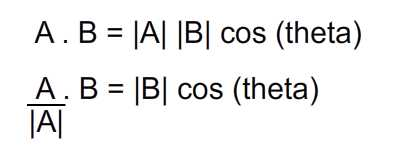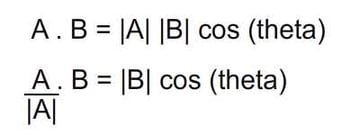And here's the perpendicular dot product definition as extracted from Wolfram: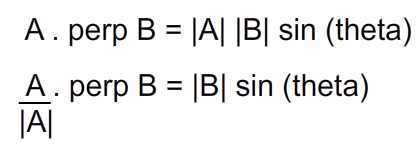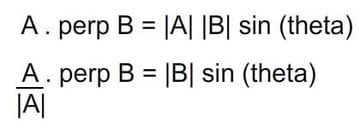## Step 2: Perpendicular Dot Product

Now to help us form a mental picture, I've prepared the image below. I'm confident at this point you are able to derive the vertical and horizontal components of a vector, so the components involving sine and cosine shouldn't be a challenge.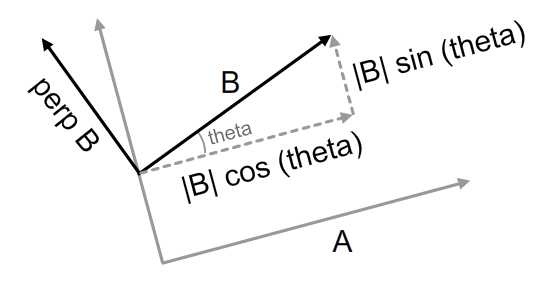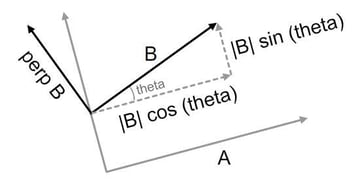Let's substitute both components with their equivalent. I have used A with a hat to represent the unit vector of A (that is, a vector that points in the same direction as A, but has a magnitude of exactly 1). Another detail is that the perpendicular of B is actually the right normal of B - more on normals next step.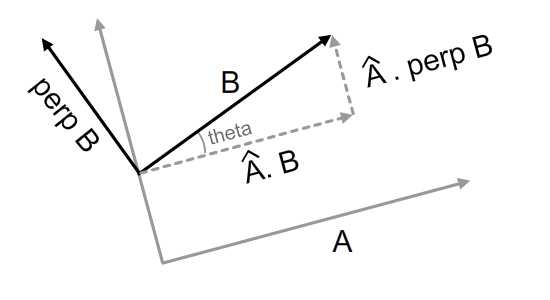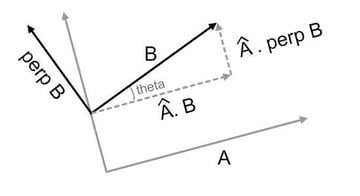From the diagram above we can see that the projection of B on A will produce |B|*cos (theta). But why would the projection of B's normal produce |B|*sin (theta)?

To better understand this, I've included a Flash demo below. Click and drag the black arrowhead. As you gently move it about, you will notice that its perpendicular axis follows as well. As they turn, the bold red lines will be also be animated. Note that these two lengths are the same - hence the equation of perpendicular dot product.

## Step 3: Normals

Normals, by definition, lie on a perpendicular line intersecting your line of interest. Let's try to imagine these lines on a geometric plane.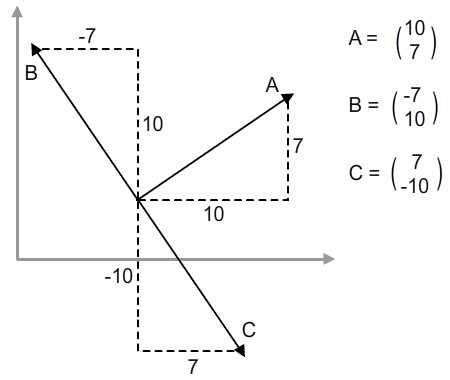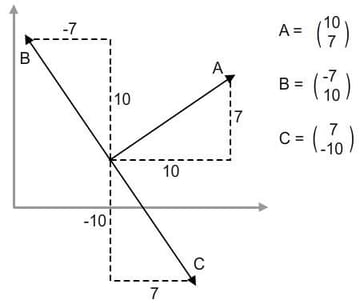The Cartesian coordinate system is used in the diagram above. B is the left normal and C is the right normal. We see that the x-component of B is negative (because it's pointing to the left) and the y-component of C is negative (because it's pointing down).

But check out the similarities between B and C. Their x and y components are the same as A's, except swizzled. The difference is only the position of the sign. So we reach a conclusion by the image below.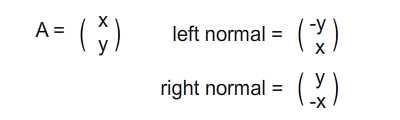Note that we are referring specifically to the Cartesian coordinate system in this example. The y-axis of Flash's coordinate space is a reflection of the one in Cartesian, resulting in a swap between the left and right normal.

## Step 4: Projecting the Point of Collision

To figure out the point of collision of vector k on plane A, we shall link up the tail of k with an arbitrary point on plane A first. For the case below, vector j is the linking vector; then we get the perpendicular projection of k and j on plane A.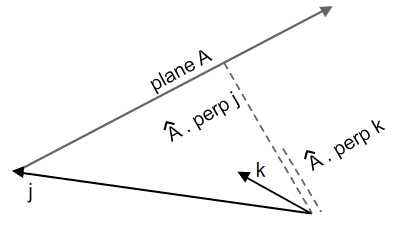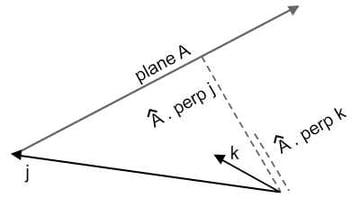The red dot on image below is the collision point. And I hope you can see the similar triangle in diagram below.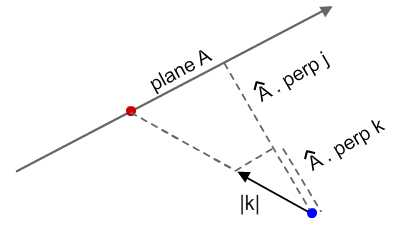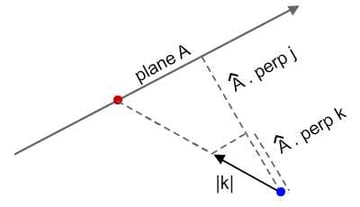• |k|, magnitude of vector k
• Length of j on perpendicular of plane A
• Length of k on perpendicular of plane A

So given the three components above, we can use the concept of ratio to deduce the length between the red and blue points. Finally, we set the magnitude of vector k to the said length and we have our collision point!

## Step 5: ActionScript Implementation

So here comes the ActionScript implementation. I've included a demo below. Try to move the arrowheads so that both lines intersect. A little black dot will mark the intersection point of the lines. Note that these segments are not necessarily intersecting, but the infinite lines they represent are.

Here's the script that does the calculations. Check out Basic.as in the source download for the full script.

## Step 6: Line Equations

So I hope the first approach I've presented was easily understood. I understand performance in getting the intersection point is important, so next I'll provide alternative approaches, although that will require some math revisions. Bear with me!

First, let's talk about line equations. There are several forms of line equation but we'll just touch two of them in this tutorial:

• General form
• Parametric form

I've included the image below to help you recall. Those interested in this can refer to this entry on Wikipedia.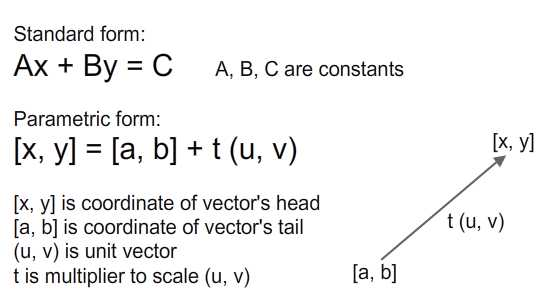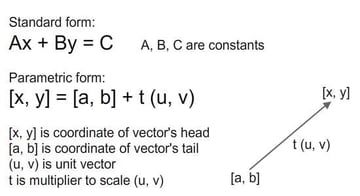## Step 7: Deriving Standard Form

Before we perform any manipulations on two line equations, we must derive these line equations first. Let's consider the scenario where we are given coordinates of two points p1 (a, b). and p2 (c, d). We can form a line equation connecting these two points from the gradients: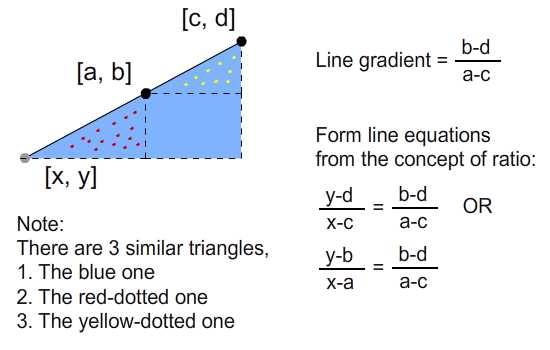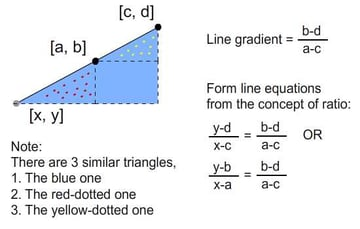Then, using this equation, we can derive the constants A, B, and C for the standard form: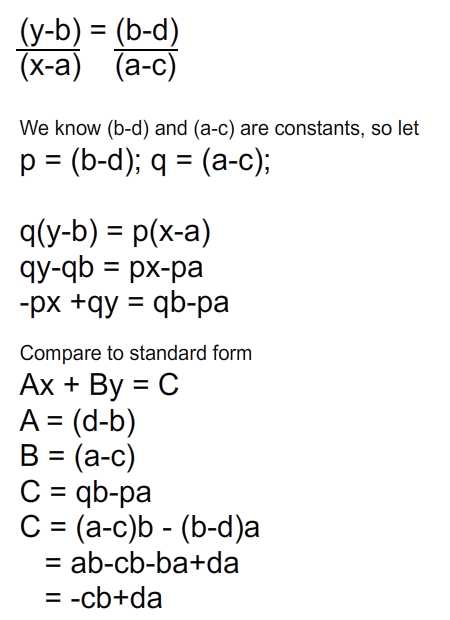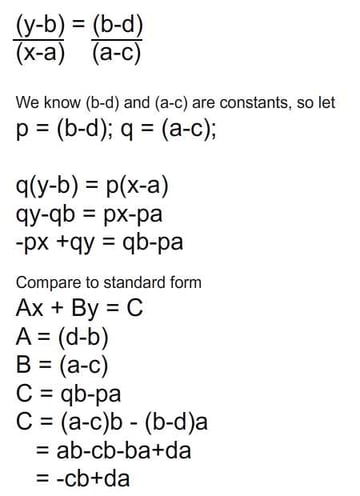Next, we can proceed to solving simultaneous line equations.

## Step 8: Solving Simultaneous Equations

Now that we can form line equations, lets proceed to take two line equations and solve them simutaneously. Given these two line equations:

• Ex + Fy = G
• Px + Qy = R

I'll table these coefficients according to the general form Ax + By = C.

 A B C E F G P Q R

In order to obtain value of y, we do the following:

1. Multiply reciprocal coefficients of x for whole equation.
2. Perform subtraction operation (from above) on both equations.
3. Rearrange obtained equation in terms of y.
 A B C Multiply by E F G P P Q R E

And we arrive at the following table.

 A B C EP FP GP PE QE RE

After we subtract two equations out, we arrive at:

• y (FP - QE) = (GP - RE), which rearranges to:
• y = (GP - RE) / (FP - QE)

Moving on to obtain x:

 A B C Multiply by E F G Q P Q R F

We arrive at the following table

 A B C EQ FQ GQ PF QF RF

After we subtract the two equations out, we arrive at:

• x (EQ - PF) = (GQ - RF), which rearranges to:
• x = (GQ - RF) / (EQ - PF)

Let's further rearrange y.

• y = (GP - RE) / (FP - QE)
• y = (GP - RE) / -(QE - FP)
• y = (RE - GP) / (QE - FP)

So we arrive at the intersection point of x and y. We notice they share the same denominator.

• x = (GQ - RF) / (EQ - PF)
• y = (RE - GP) / (QE - FP)

Now that we have worked out the math operations and gotten the result, just pluck values in and we have the intersection point.

## Step 9: Applying Onto Actionscript

Here's the Actionscript implementation. So all vector operations are reduced to simple arithmetic but it'll require some algebra workings initially.

Of course it's the same result as previous demo, just with less maths involved, and with no use of the Vector2D class.

## Step 10: Matrix Alternative

Another alternative to solving this problem is by the use of matrix math. Again, I invite interested readers to dive into Prof. Wildberger's lecture on equations of lines. Here, we'll just breeze through the concept quickly.

According to Prof Wildberger, there are two frameworks we can adopt:

• The Cartesian framework
• The parameterised vector framework

Let's go through the Cartesian one first. Check out the image below.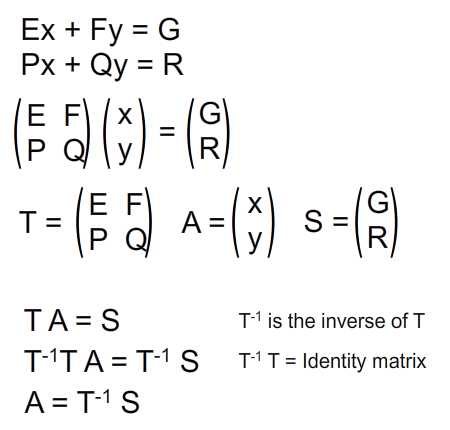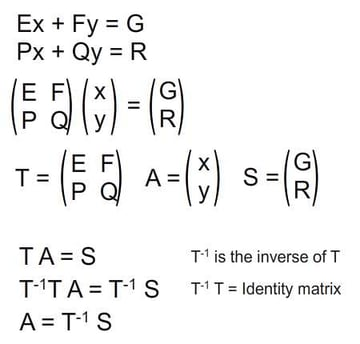Note that matrix T and S contain constant values. What's left unknown is A. So rearranging the matrix equation in terms of A will give us the result. However, we must get the inverse matrix of T.

## Step 11: Implementing With AS3

Here's the implementation of the above with ActionScript:

## Step 12: Parametric Form

Lastly, there's the parametric form of the line equation, and we shall attempt to solve it through matrix math again.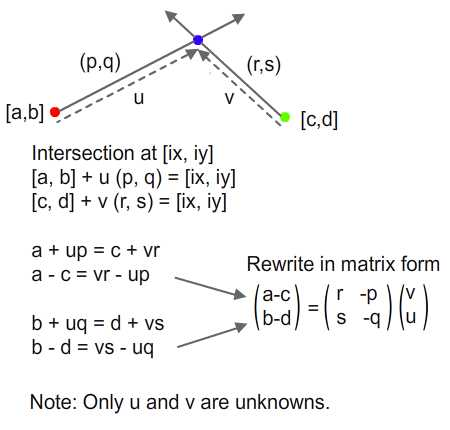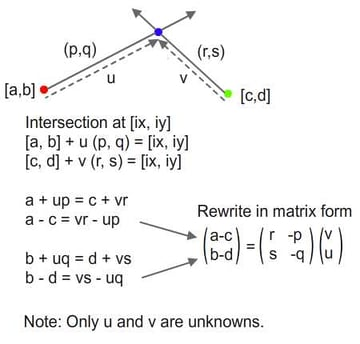We'd like to get the intersection point. Given all information except for u and v which we attempt to find, we shall rewrite both the equations into matrix form and solve them.

## Step 13: Matrix Manipulation

So again, we perform matrix manipulations to arrive at our result.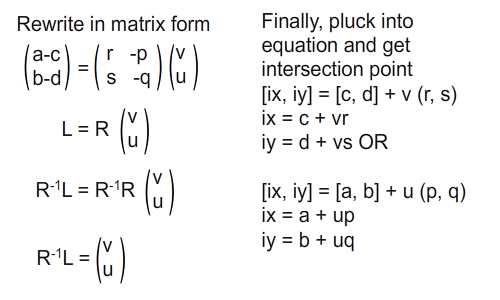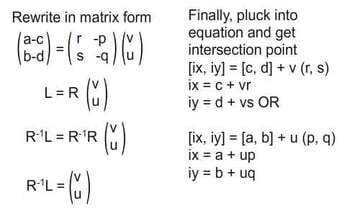## Step 14: Implementing With AS3

So here's the implementation of the matrix form:

## Step 15: Performance

We've covered four approaches to solve this little problem. So what about performance? Well I think I'll just leave this issue to readers to judge, although I believe the difference is negligible. Feel free to utilise this performance testing harness from Grant Skinner.

So now that we've gotten this understanding, what's next? Apply it!

## Step 16: Predicting Collision Time

Assume a particle is moving in a path bound to collide with a wall. We can calculate the time to impact by the simple equation of:

Velocity = Displacement / Time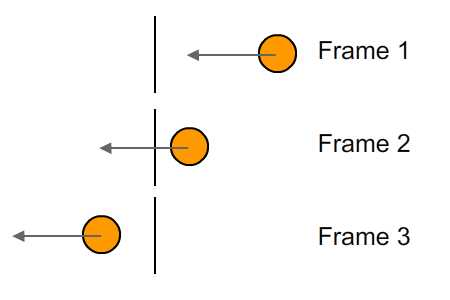Imagine you are inside of this orange round particle and for each passing frame and announcement is made on the time to collide with wall. You'll hear:

"Time to impact: 1.5 frames" - Frame 1

"Time to impact: 0.5 frames" - Frame 2

"Time to impact: -0.5 frames" - Frame 3

When we reach frame 3, collision with line has already happened (as indicated by the negative sign). You have to rewind time in order to reach the point of collision. Obviously collision should happen some time between Frames 2 and 3, but Flash moves in single frame increments. So if collision happened halfway between frames, a flip of the sign to negative will indicate that the collision has already happened.

## Step 17: Negative Time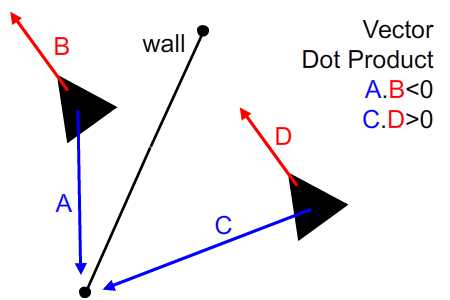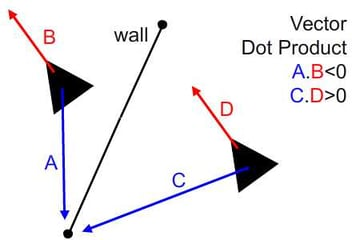In order to get negative time, we'll use the vector dot product. We know that when we have two vectors and the direction of one is not within 90 degrees either side of the other, they will produce a negative dot product. Also, the dot product is a measure of how parallel two vectors are. So when collision has already happened, the velocity and direction of a vector to a point on wall will be negative - and vice versa.

## Step 18: ActionScript Implementation

So here's the script (included in CollisionTime.as). I've also added collision detection within line segment here. For those who find it unfamiliar, do refer to my tutorial on collision detection between a circle and a line segment, Step 6. And for help on steering ships, here's another reference.

## Step 19: Final Result

So here's a demo of what you will arrive at. Use the left and right arrow keys to steer the ship (triangle), and press Up to boost speed temporarily. If the predicted future collision point is on the wall (the line), a red dot will be painted on it. For collision that has "already" happened, a red dot will still be painted but slightly transparent. You may also drag the black dots either side of the wall to move it.

## Conclusion

So I hope this tutorial has been informative. Do share if you've actually applied this idea elsewhere from the one I've mentioned. I'm planning a brief write-up on applying it to paint laser targets - what do you think? Thanks for reading and let me know if there are any errors.